# NCERT Exemplar Class 12 Chemistry Solutions for Chapter 3 - Electrochemistry Solutions

NCERT Class 12 Chemistry Exemplar Solutions for Chapter 3 Electrochemistry aid in learning and testing students’ comprehension, information recall, analytical thinking and problem-solving abilities. This exemplar solution is the most comprehensive study material students rely on to practise different types of questions to prepare well for their Class 12 and entrance examinations.

The NCERT Class 12 Chemistry Exemplar for Chapter 3 PDF has questions and answers from the exemplar book, together with Class 12 Chemistry extra questions, Electrochemistry Class 12 problems, Chemistry problems and solutions for Class 12, MCQs, exercises, assignments and important questions from the previous year Class 12  question papers and sample papers.

## Download the PDF of NCERT Exemplar Solutions for Class 12 Chemistry Chapter 3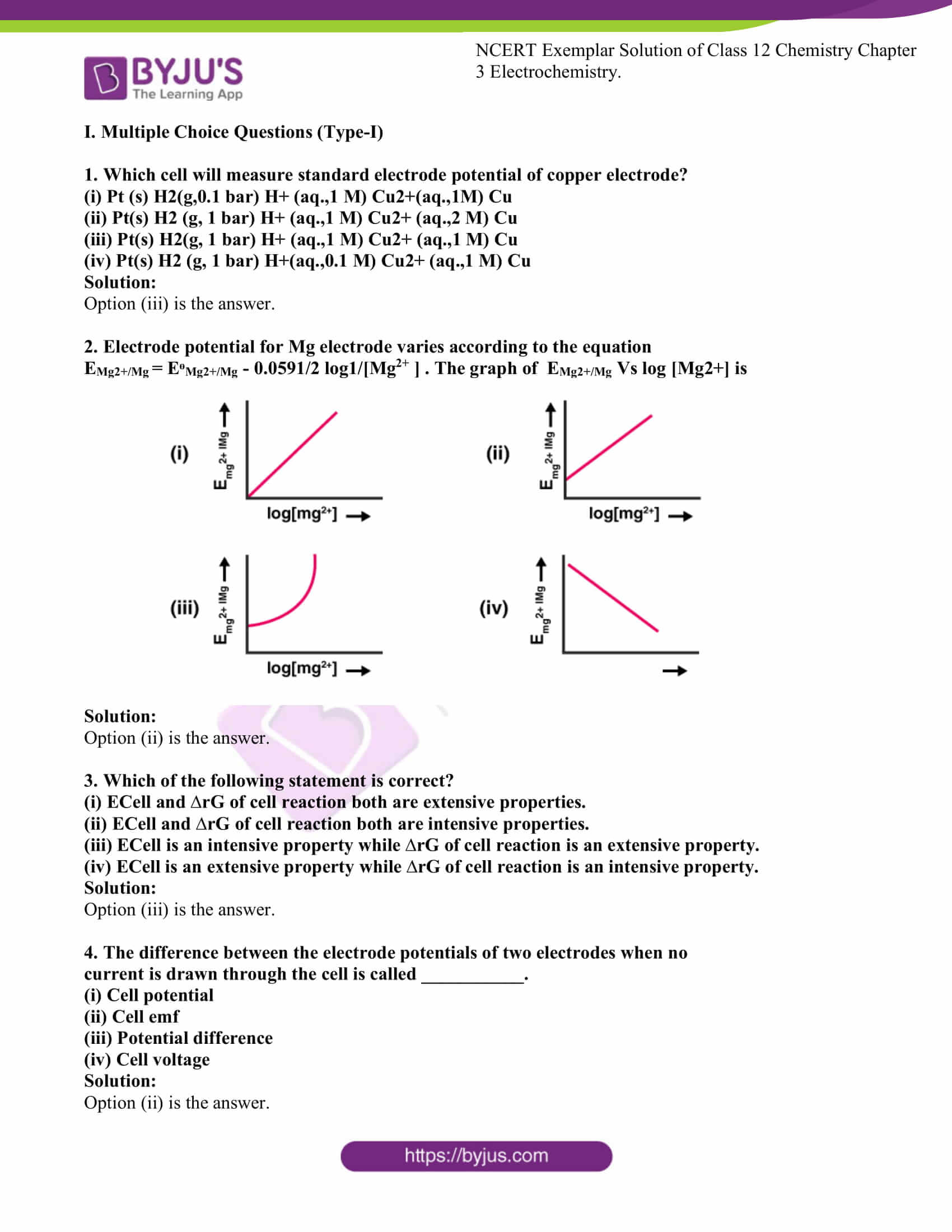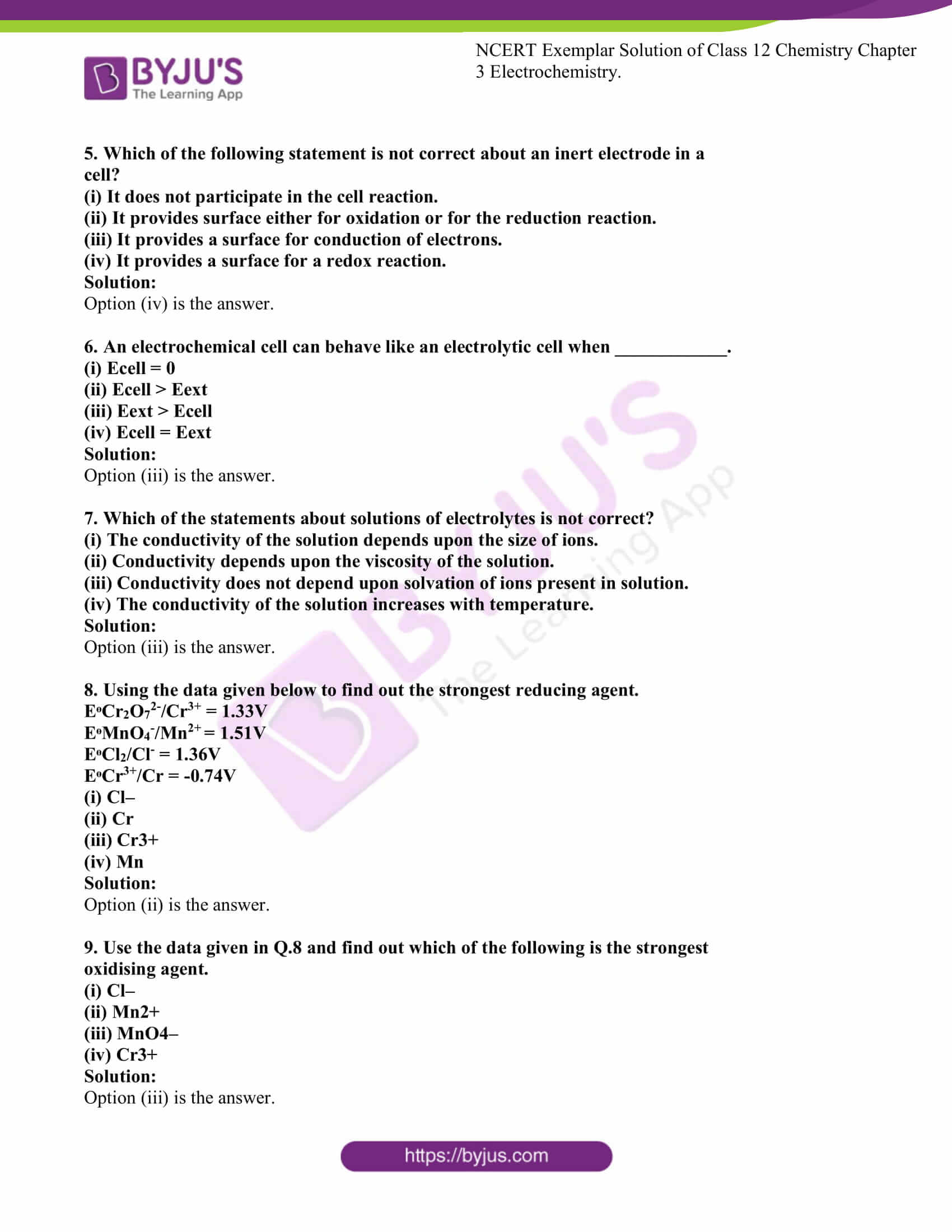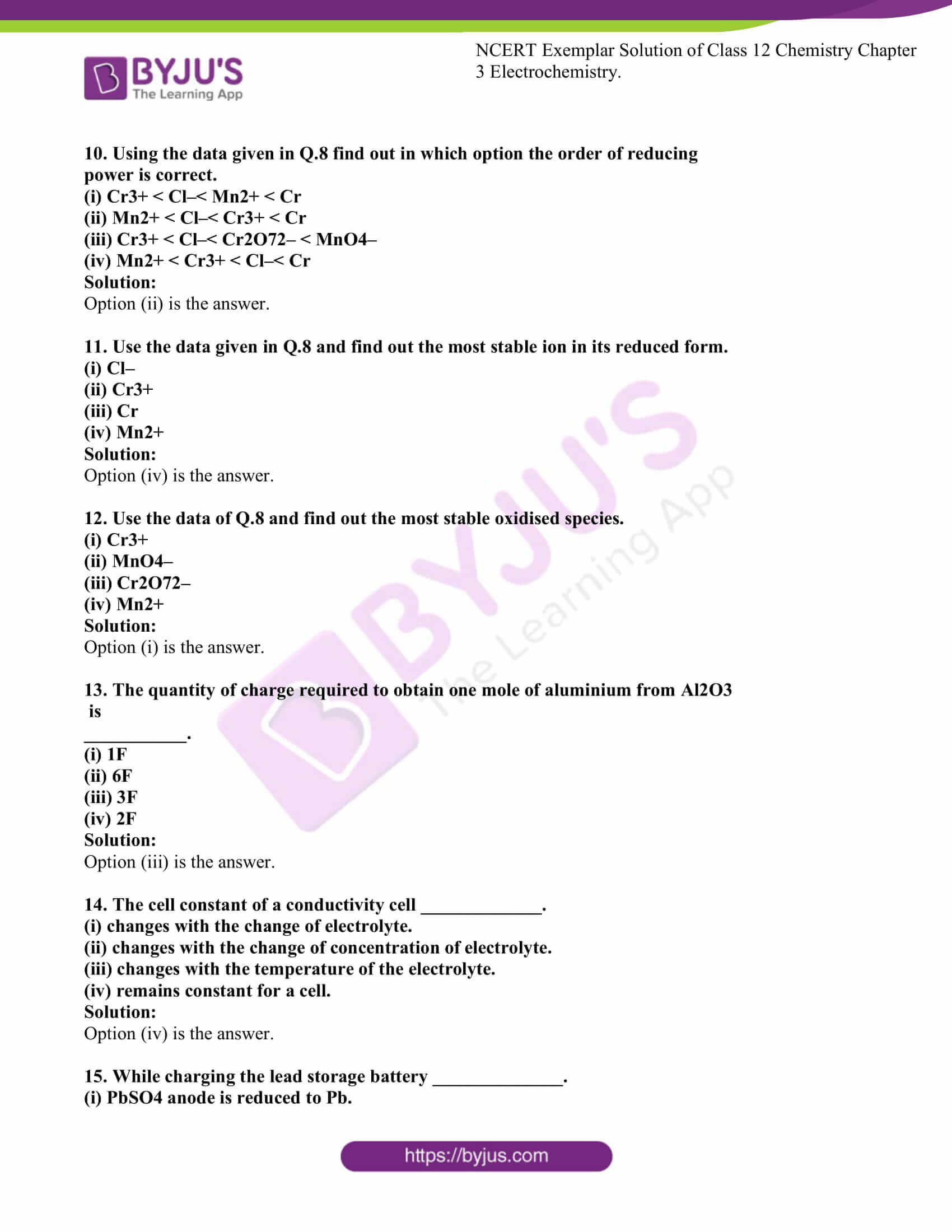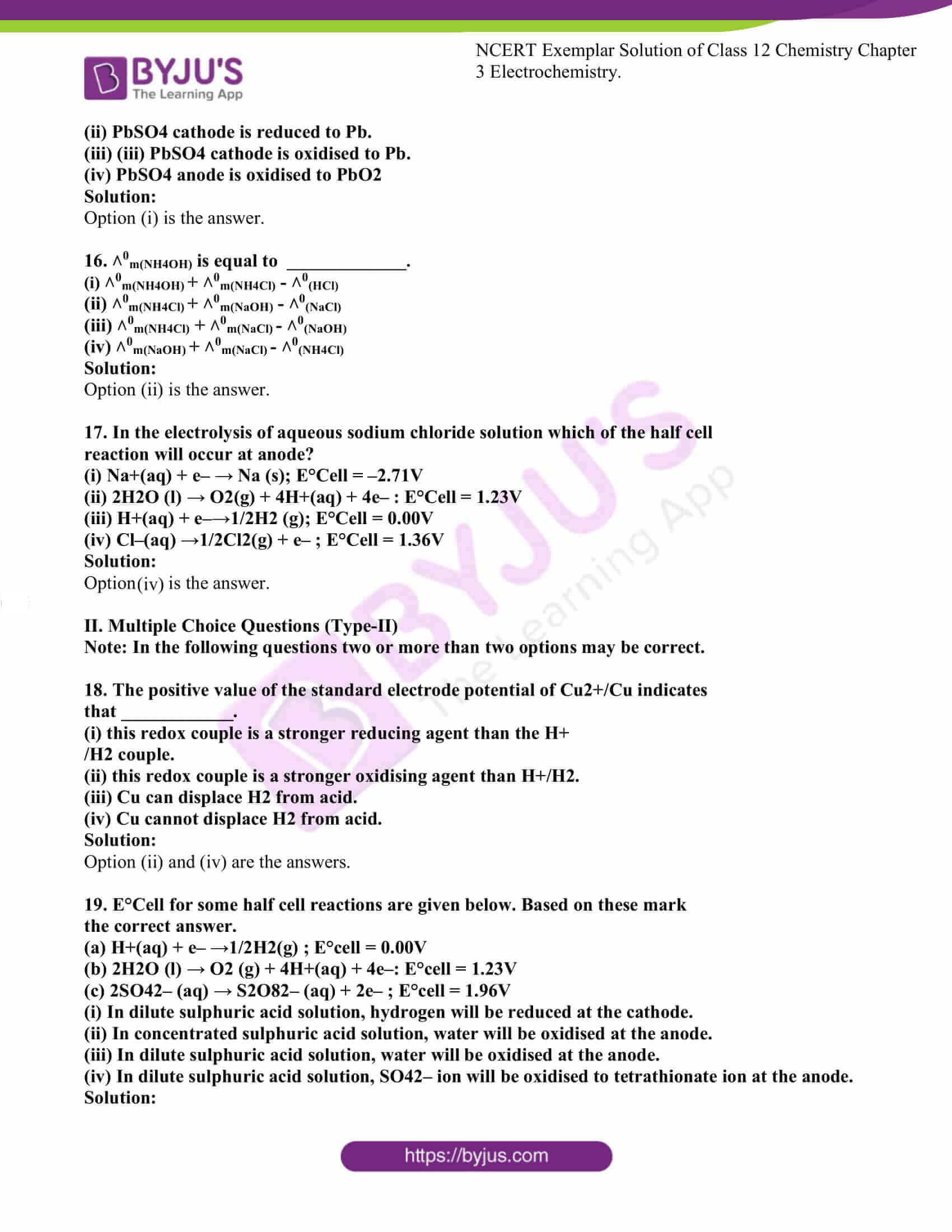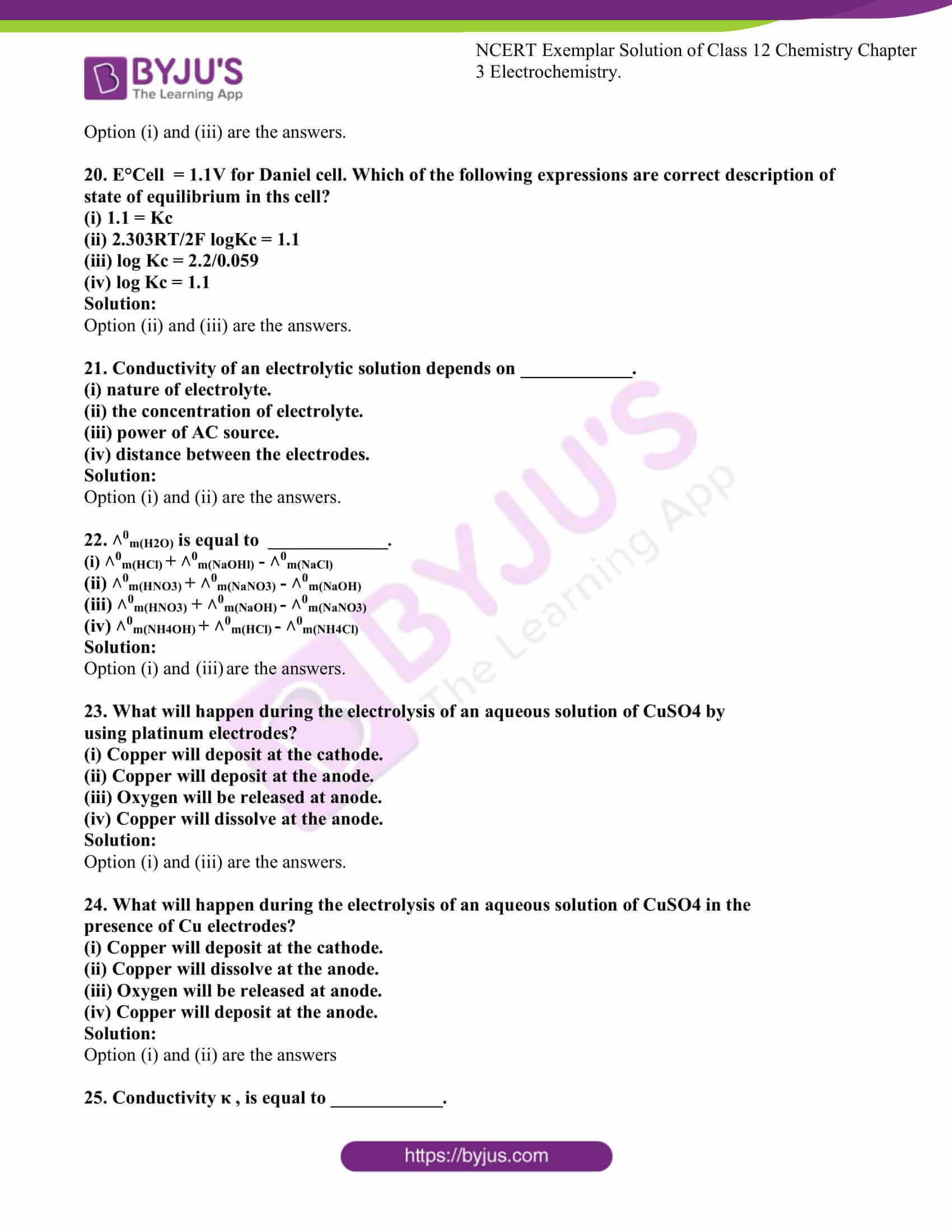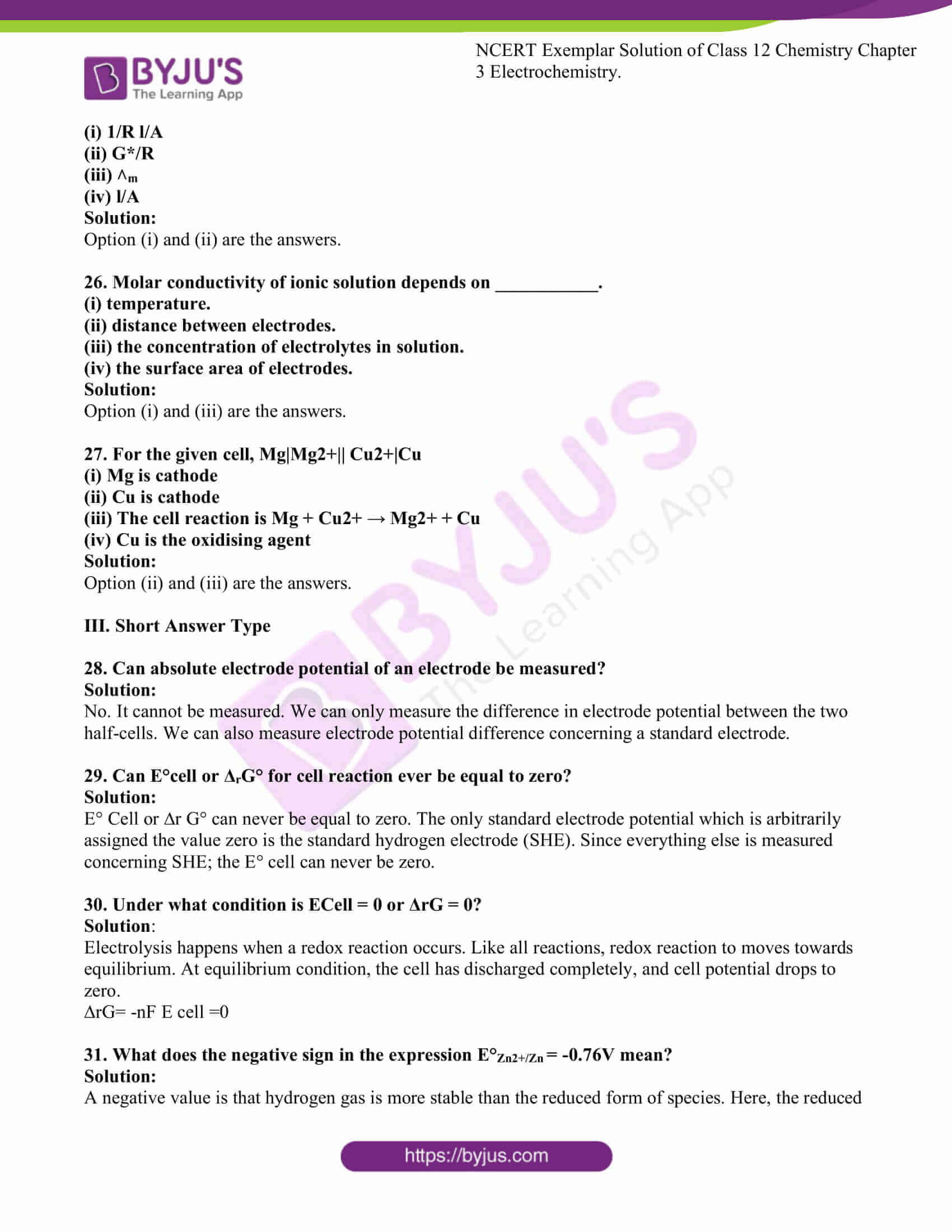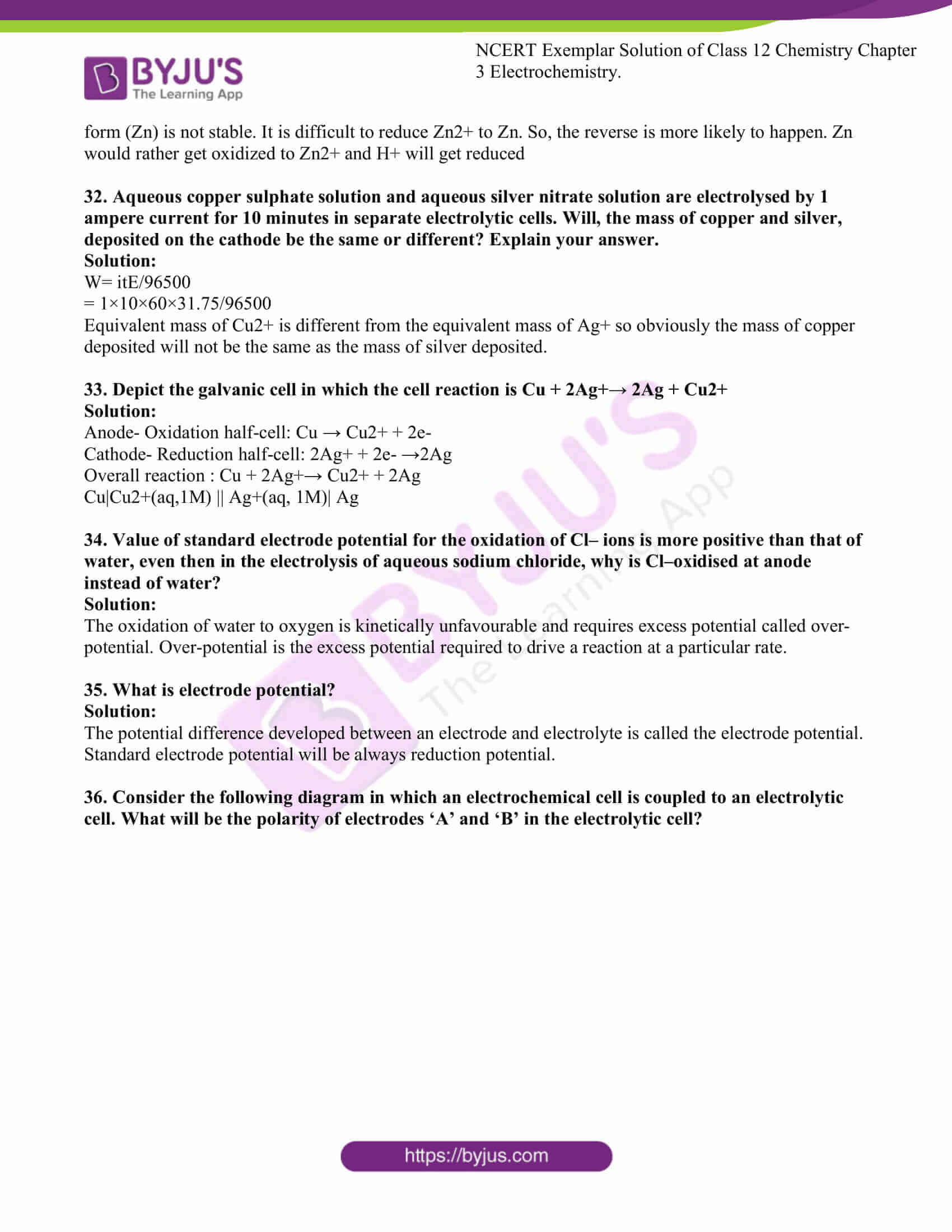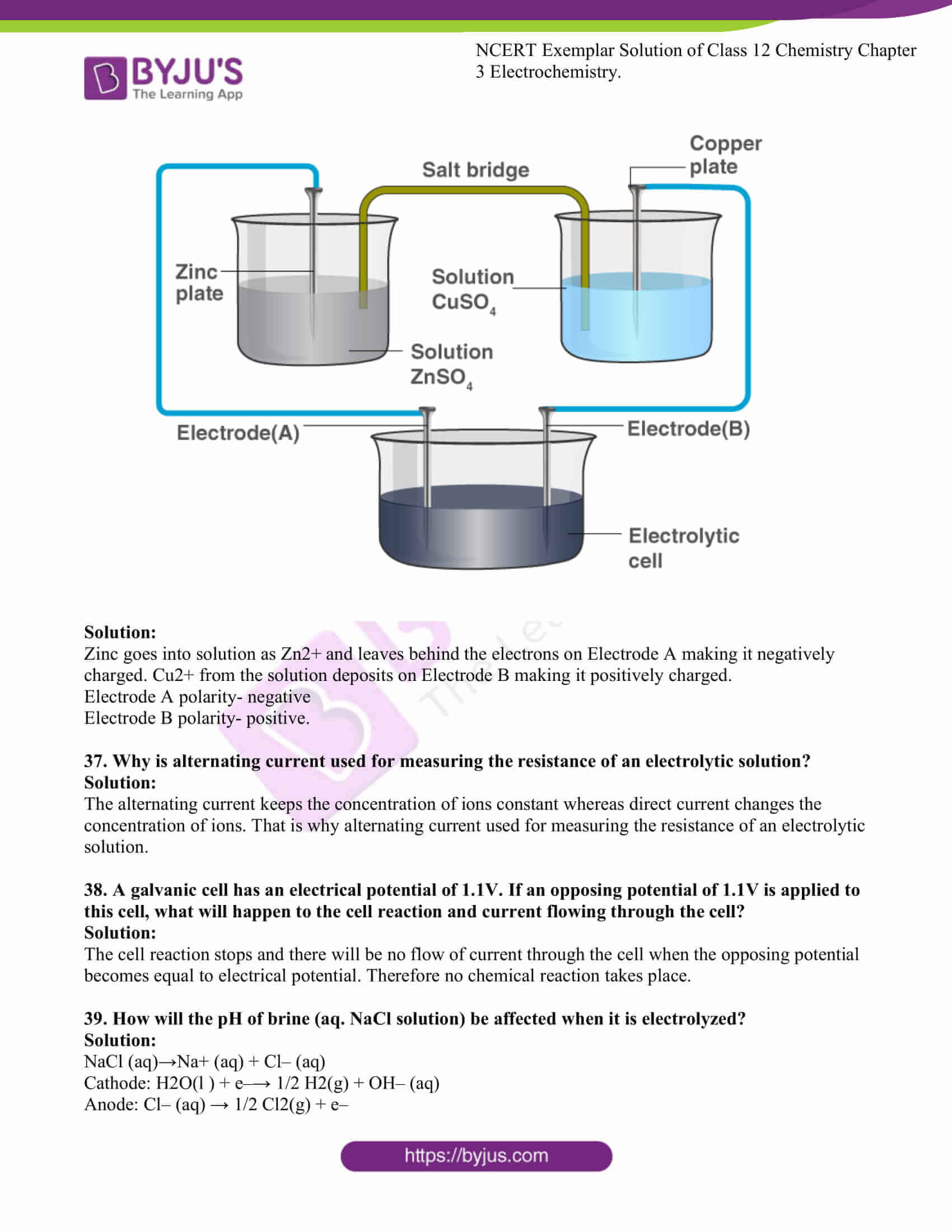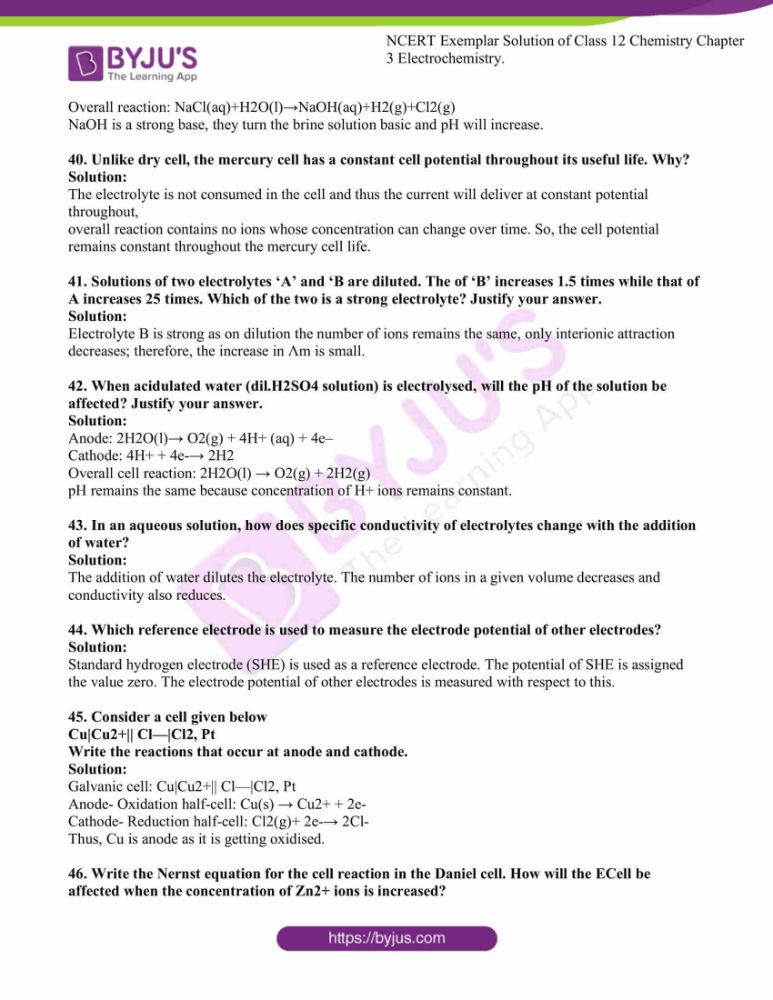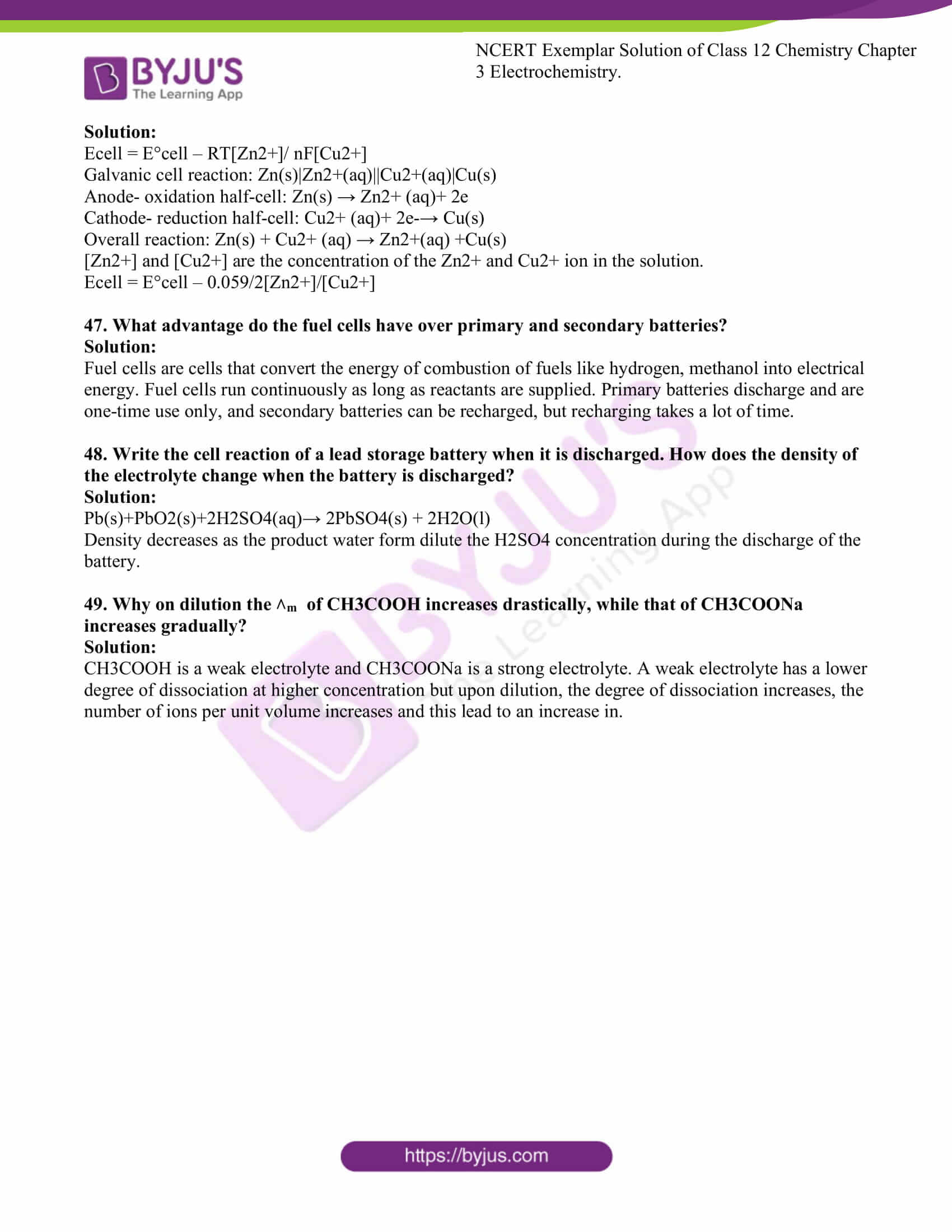### Access Solutions to the NCERT Exemplar Class 12 Chemistry Chapter 3

I. Multiple Choice Questions (Type-I)

1. Which cell will measure standard electrode potential of copper electrode?

(i) Pt (s) H2(g,0.1 bar) H+ (aq.,1 M) Cu2+(aq.,1M) Cu

(ii) Pt(s) H2 (g, 1 bar) H+ (aq.,1 M) Cu2+ (aq.,2 M) Cu

(iii) Pt(s) H2(g, 1 bar) H+ (aq.,1 M) Cu2+ (aq.,1 M) Cu

(iv) Pt(s) H2 (g, 1 bar) H+(aq.,0.1 M) Cu2+ (aq.,1 M) Cu

Solution:

2. Electrode potential for Mg electrode varies according to the equation

EMg2+/Mg = EᶱMg2+/Mg – 0.0591/2 log1/[Mg2+ ] . The graph of EMg2+/Mg Vs log [Mg2+] is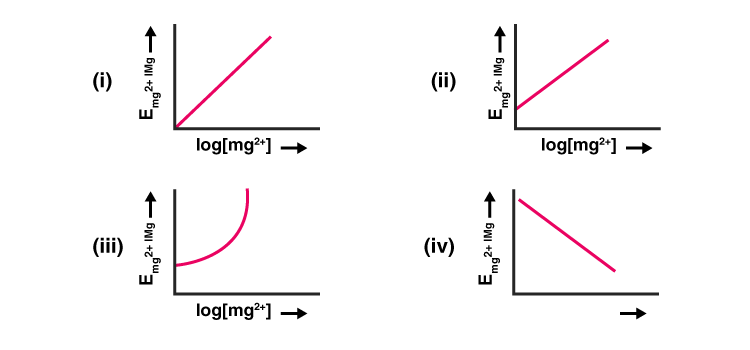Solution:

3. Which of the following statement is correct?

(i) ECell and ∆rG of cell reaction both are extensive properties.

(ii) ECell and ∆rG of cell reaction both are intensive properties.

(iii) ECell is an intensive property while ∆rG of cell reaction is an extensive property.

(iv) ECell is an extensive property while ∆rG of cell reaction is an intensive property.

Solution:

4. The difference between the electrode potentials of two electrodes when no

current is drawn through the cell is called ___________.

(i) Cell potential

(ii) Cell emf

(iii) Potential difference

(iv) Cell voltage

Solution:

5. Which of the following statement is not correct about an inert electrode in a

cell?

(i) It does not participate in the cell reaction.

(ii) It provides surface either for oxidation or for the reduction reaction.

(iii) It provides a surface for conduction of electrons.

(iv) It provides a surface for a redox reaction.

Solution:

6. An electrochemical cell can behave like an electrolytic cell when ____________.

(i) Ecell = 0

(ii) Ecell > Eext

(iii) Eext > Ecell

(iv) Ecell = Eext

Solution:

7. Which of the statements about solutions of electrolytes is not correct?

(i) The conductivity of the solution depends upon the size of ions.

(ii) Conductivity depends upon the viscosity of the solution.

(iii) Conductivity does not depend upon solvation of ions present in solution.

(iv) The conductivity of the solution increases with temperature.

Solution:

8. Using the data given below to find out the strongest reducing agent.

EᶱCr2O72-/Cr3+ = 1.33V

EᶱMnO4/Mn2+ = 1.51V

EᶱCl2/Cl = 1.36V

EᶱCr3+/Cr = -0.74V

(i) Cl–

(ii) Cr

(iii) Cr3+

(iv) Mn

Solution:

9. Use the data given in Q.8 and find out which of the following is the strongest

oxidising agent.

(i) Cl–

(ii) Mn2+

(iii) MnO4–

(iv) Cr3+

Solution:

10. Using the data given in Q.8 find out in which option the order of reducing

power is correct.

(i) Cr3+ < Cl–< Mn2+ < Cr

(ii) Mn2+ < Cl–< Cr3+ < Cr

(iii) Cr3+ < Cl–< Cr2O72– < MnO4–

(iv) Mn2+ < Cr3+ < Cl–< Cr

Solution:

11. Use the data given in Q.8 and find out the most stable ion in its reduced form.

(i) Cl–

(ii) Cr3+

(iii) Cr

(iv) Mn2+

Solution:

12. Use the data of Q.8 and find out the most stable oxidised species.

(i) Cr3+

(ii) MnO4–

(iii) Cr2O72–

(iv) Mn2+

Solution:

13. The quantity of charge required to obtain one mole of aluminium from Al2O3

is

___________.

(i) 1F

(ii) 6F

(iii) 3F

(iv) 2F

Solution:

14. The cell constant of a conductivity cell _____________.

(i) changes with the change of electrolyte.

(ii) changes with the change of concentration of electrolyte.

(iii) changes with the temperature of the electrolyte.

(iv) remains constant for a cell.

Solution:

15. While charging the lead storage battery ______________.

(i) PbSO4 anode is reduced to Pb.

(ii) PbSO4 cathode is reduced to Pb.(iii) (iii) PbSO4 cathode is oxidised to Pb.

(iv) PbSO4 anode is oxidised to PbO2

Solution:

16. ˄0m(NH4OH) is equal to ______________.

(i) ˄0m(NH4OH) + ˄0m(NH4Cl) – ˄0(HCl)

(ii) ˄0m(NH4Cl) + ˄0m(NaOH) – ˄0(NaCl)

(iii) ˄0m(NH4Cl) + ˄0m(NaCl) – ˄0(NaOH)

(iv) ˄0m(NaOH) + ˄0m(NaCl) – ˄0(NH4Cl)

Solution:

17. In the electrolysis of aqueous sodium chloride solution which of the half cell

reaction will occur at anode?

(i) Na+(aq) + e– → Na (s); E°Cell = –2.71V

(ii) 2H2O (l) → O2(g) + 4H+(aq) + 4e– : E°Cell = 1.23V

(iii) H+(aq) + e–→1/2H2 (g); E°Cell = 0.00V

(iv) Cl–(aq) →1/2Cl2(g) + e– ; E°Cell = 1.36V

Solution:

II. Multiple Choice Questions (Type-II)

Note: In the following questions two or more than two options may be correct.

18. The positive value of the standard electrode potential of Cu2+/Cu indicates

that ____________.

(i) this redox couple is a stronger reducing agent than the H+

/H2 couple.

(ii) this redox couple is a stronger oxidising agent than H+/H2.

(iii) Cu can displace H2 from acid.

(iv) Cu cannot displace H2 from acid.

Solution:

Option (ii) and (iv) are the answers.

19. E°Cell for some half cell reactions are given below. Based on these mark

(a) H+(aq) + e– →1/2H2(g) ; E°cell = 0.00V

(b) 2H2O (l) → O2 (g) + 4H+(aq) + 4e–: E°cell = 1.23V

(c) 2SO42– (aq) → S2O82– (aq) + 2e– ; E°cell = 1.96V

(i) In dilute sulphuric acid solution, hydrogen will be reduced at the cathode.

(ii) In concentrated sulphuric acid solution, water will be oxidised at the anode.

(iii) In dilute sulphuric acid solution, water will be oxidised at the anode.

(iv) In dilute sulphuric acid solution, SO42– ion will be oxidised to tetrathionate ion at the anode.

Solution:

Option (i) and (iii) are the answers.

20. E°Cell = 1.1V for Daniel cell. Which of the following expressions are correct description of state of equilibrium in ths cell?

(i) 1.1 = Kc

(ii) 2.303RT/2F logKc = 1.1

(iii) log Kc = 2.2/0.059

(iv) log Kc = 1.1

Solution:

Option (ii) and (iii) are the answers.

21. Conductivity of an electrolytic solution depends on ____________.

(i) nature of electrolyte.

(ii) the concentration of electrolyte.

(iii) power of AC source.

(iv) distance between the electrodes.

Solution:

Option (i) and (ii) are the answers.

22. ˄0m(H2O) is equal to ______________.

(i) ˄0m(HCl) + ˄0m(NaOHl) – ˄0m(NaCl)

(ii) ˄0m(HNO3) + ˄0m(NaNO3) – ˄0m(NaOH)

(iii) ˄0m(HNO3) + ˄0m(NaOH) – ˄0m(NaNO3)

(iv) ˄0m(NH4OH) + ˄0m(HCl) – ˄0m(NH4Cl)

Solution:

Option (i) and (iii) are the answers.

23. What will happen during the electrolysis of an aqueous solution of CuSO4 by

using platinum electrodes?

(i) Copper will deposit at the cathode.

(ii) Copper will deposit at the anode.

(iii) Oxygen will be released at anode.

(iv) Copper will dissolve at the anode.

Solution:

Option (i) and (iii) are the answers.

24. What will happen during the electrolysis of an aqueous solution of CuSO4 in the

presence of Cu electrodes?

(i) Copper will deposit at the cathode.

(ii) Copper will dissolve at the anode.

(iii) Oxygen will be released at anode.

(iv) Copper will deposit at the anode.

Solution:

Option (i) and (ii) are the answers

25. Conductivity κ , is equal to ____________.

(i) 1/R l/A

(ii) G*/R

(iii) ˄m

(iv) l/A

Solution:

Option (i) and (ii) are the answers.

26. Molar conductivity of ionic solution depends on ___________.

(i) temperature.

(ii) distance between electrodes.

(iii) the concentration of electrolytes in solution.

(iv) the surface area of electrodes.

Solution:

Option (i) and (iii) are the answers.

27. For the given cell, Mg|Mg2+|| Cu2+|Cu

(i) Mg is cathode

(ii) Cu is cathode

(iii) The cell reaction is Mg + Cu2+ → Mg2+ + Cu

(iv) Cu is the oxidising agentSolution:

Option (ii) and (iii) are the answers.

28. Can absolute electrode potential of an electrode be measured?

Solution:

No. It cannot be measured. We can only measure the difference in electrode potential between the two half-cells. We can also measure electrode potential difference concerning a standard electrode.

29. Can E°cell or ΔrG° for cell reaction ever be equal to zero?

Solution:

E° Cell or ∆r G° can never be equal to zero. The only standard electrode potential which is arbitrarily assigned the value zero is the standard hydrogen electrode (SHE). Since everything else is measured concerning SHE; the E° cell can never be zero.

30. Under what condition is ECell = 0 or ΔrG = 0?

Solution:

Electrolysis happens when a redox reaction occurs. Like all reactions, redox reaction to moves towards equilibrium. At equilibrium condition, the cell has discharged completely, and cell potential drops to zero.

∆rG= -nF E cell =0

31. What does the negative sign in the expression E°Zn2+/Zn = -0.76V mean?

Solution:

A negative value is that hydrogen gas is more stable than the reduced form of species. Here, the reduced form (Zn) is not stable. It is difficult to reduce Zn2+ to Zn. So, the reverse is more likely to happen. Zn would rather get oxidized to Zn2+ and H+ will get reduced

32. Aqueous copper sulphate solution and aqueous silver nitrate solution are electrolysed by 1 ampere current for 10 minutes in separate electrolytic cells. Will, the mass of copper and silver, deposited on the cathode be the same or different? Explain your answer.

Solution:

W= itE/96500

= 1×10×60×31.75/96500

Equivalent mass of Cu2+ is different from the equivalent mass of Ag+ so obviously the mass of copper deposited will not be the same as the mass of silver deposited.

33. Depict the galvanic cell in which the cell reaction is Cu + 2Ag+→ 2Ag + Cu2+

Solution:

Anode- Oxidation half-cell: Cu → Cu2+ + 2e-

Cathode- Reduction half-cell: 2Ag+ + 2e- →2Ag

Overall reaction : Cu + 2Ag+→ Cu2+ + 2Ag

Cu|Cu2+(aq,1M) || Ag+(aq, 1M)| Ag

34. Value of standard electrode potential for the oxidation of Cl– ions is more positive than that of water, even then in the electrolysis of aqueous sodium chloride, why is Cl–oxidised at anode instead of water?

Solution:

The oxidation of water to oxygen is kinetically unfavourable and requires excess potential called over-potential. Over-potential is the excess potential required to drive a reaction at a particular rate.

35. What is electrode potential?

Solution:

The potential difference developed between an electrode and electrolyte is called the electrode potential.

Standard electrode potential will be always reduction potential.

36. Consider the following diagram in which an electrochemical cell is coupled to an electrolytic cell. What will be the polarity of electrodes ‘A’ and ‘B’ in the electrolytic cell?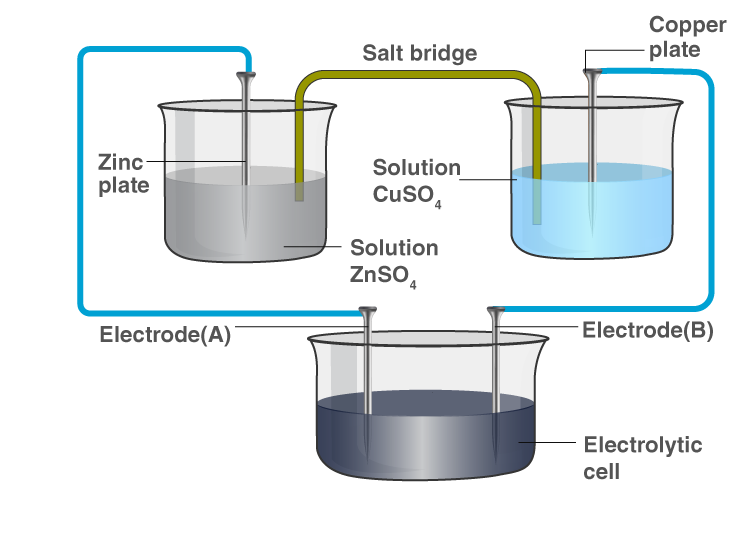Solution:

Zinc goes into solution as Zn2+ and leaves behind the electrons on Electrode A making it negatively charged. Cu2+ from the solution deposits on Electrode B making it positively charged.

Electrode A polarity- negative

Electrode B polarity- positive.

37. Why is alternating current used for measuring the resistance of an electrolytic solution?

Solution:

The alternating current keeps the concentration of ions constant whereas direct current changes the concentration of ions. That is why alternating current used for measuring the resistance of an electrolytic solution.

38. A galvanic cell has an electrical potential of 1.1V. If an opposing potential of 1.1V is applied to this cell, what will happen to the cell reaction and current flowing through the cell?

Solution:

The cell reaction stops and there will be no flow of current through the cell when the opposing potential becomes equal to electrical potential. Therefore no chemical reaction takes place.

39. How will the pH of brine (aq. NaCl solution) be affected when it is electrolyzed?

Solution:

NaCl (aq)→Na+ (aq) + Cl– (aq)

Cathode: H2O(l ) + e–→ 1/2 H2(g) + OH– (aq)

Anode: Cl– (aq) → 1/2 Cl2(g) + e–

Overall reaction: NaCl(aq)+H2O(l)→NaOH(aq)+H2(g)+Cl2(g)

NaOH is a strong base, they turn the brine solution basic and pH will increase.

40. Unlike dry cell, the mercury cell has a constant cell potential throughout its useful life. Why?

Solution:

The electrolyte is not consumed in the cell and thus the current will deliver at constant potential throughout,

overall reaction contains no ions whose concentration can change over time. So, the cell potential remains constant throughout the mercury cell life.

41. Solutions of two electrolytes ‘A’ and ‘B are diluted. The of ‘B’ increases 1.5 times while that of A increases 25 times. Which of the two is a strong electrolyte? Justify your answer.

Solution:

Electrolyte B is strong as on dilution the number of ions remains the same, only interionic attraction decreases; therefore, the increase in Λm is small.

42. When acidulated water (dil.H2SO4 solution) is electrolysed, will the pH of the solution be affected? Justify your answer.

Solution:

Anode: 2H2O(l)→ O2(g) + 4H+ (aq) + 4e–

Cathode: 4H+ + 4e-→ 2H2

Overall cell reaction: 2H2O(l) → O2(g) + 2H2(g)

pH remains the same because concentration of H+ ions remains constant.

43. In an aqueous solution, how does specific conductivity of electrolytes change with the addition of water?

Solution:

The addition of water dilutes the electrolyte. The number of ions in a given volume decreases and conductivity also reduces.

44. Which reference electrode is used to measure the electrode potential of other electrodes?

Solution:

Standard hydrogen electrode (SHE) is used as a reference electrode. The potential of SHE is assigned the value zero. The electrode potential of other electrodes is measured with respect to this.

45. Consider a cell given below

Cu|Cu2+|| Cl—|Cl2, Pt

Write the reactions that occur at anode and cathode.

Solution:

Galvanic cell: Cu|Cu2+|| Cl—|Cl2, Pt

Anode- Oxidation half-cell: Cu(s) → Cu2+ + 2e-

Cathode- Reduction half-cell: Cl2(g)+ 2e-→ 2Cl-

Thus, Cu is anode as it is getting oxidised.

46. Write the Nernst equation for the cell reaction in the Daniel cell. How will the ECell be affected when the concentration of Zn2+ ions is increased?

Solution:

Ecell = E°cell – RT[Zn2+]/ nF[Cu2+]

Galvanic cell reaction: Zn(s)|Zn2+(aq)||Cu2+(aq)|Cu(s)

Anode- oxidation half-cell: Zn(s) → Zn2+ (aq)+ 2e

Cathode- reduction half-cell: Cu2+ (aq)+ 2e-→ Cu(s)

Overall reaction: Zn(s) + Cu2+ (aq) → Zn2+(aq) +Cu(s)

[Zn2+] and [Cu2+] are the concentration of the Zn2+ and Cu2+ ion in the solution.

Ecell = E°cell – 0.059/2[Zn2+]/[Cu2+]

47. What advantage do the fuel cells have over primary and secondary batteries?

Solution:

Fuel cells are cells that convert the energy of combustion of fuels like hydrogen, methanol into electrical energy. Fuel cells run continuously as long as reactants are supplied. Primary batteries discharge and are one-time use only, and secondary batteries can be recharged, but recharging takes a lot of time.

48. Write the cell reaction of a lead storage battery when it is discharged. How does the density of the electrolyte change when the battery is discharged?

Solution:

Pb(s)+PbO2(s)+2H2SO4(aq)→ 2PbSO4(s) + 2H2O(l)

Density decreases as the product water form dilute the H2SO4 concentration during the discharge of the battery.

49. Why on dilution the ˄m of CH3COOH increases drastically, while that of CH3COONa increases gradually?

Solution:

CH3COOH is a weak electrolyte and CH3COONa is a strong electrolyte. A weak electrolyte has a lower degree of dissociation at higher concentration but upon dilution, the degree of dissociation increases, the number of ions per unit volume increases, and this lead to an increase in.

### Class 12 Chemistry NCERT Exemplar Solutions on Electrochemistry

Before solving the NCERT Exemplar Class 12 Chemistry Chapter 3 Electrochemistry, students must learn what electrochemistry is. Electrochemistry is the study of chemical processes that cause electrons to move. After studying the chapter, students will understand more about Kohlrausch law and learn its applications and corrosion.

#### The Important Concepts of Class 12 Chemistry Chapter 3 Electrochemistry

1. Electrochemical Cells
2. Galvanic Cells
• Measurement of Electrode Potential
3. Nernst Equation
• Equilibrium Constant from Nernst Equation
• Electrochemical Cell and Gibbs Energy of Reaction
4. The conductance of Electrolytic Solutions
• Measurement of the Conductivity of Ionic Solutions
• Variation of Conductivity and Molar Conductivity with Concentration
5. Electrolytic Cells and Electrolysis
• Products of Electrolysis
6. Batteries
• Primary Batteries
• Secondary Batteries
7. Fuel Cells
8. Corrosion

Access the NCERT exemplar questions from the given link.

### Why Opt for BYJU’S

The NCERT Class 12 Chemistry Exemplar for Chapter 3 is prepared by Chemistry experts at BYJU’S, as per the latest CBSE syllabus for 2023-24. Solutions to the questions help you describe an electrochemical cell and differentiate between galvanic and electrolytic cells. You will also study the application of the Nernst equation for calculating the emf of a galvanic cell and define the standard potential of the cell.